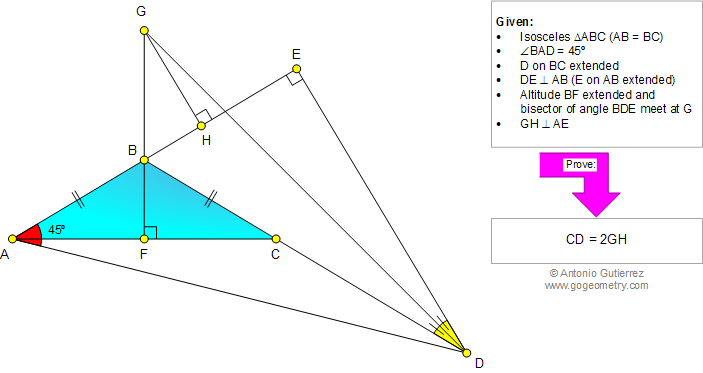# Geometry Problem 958. Isosceles Triangle, Altitude, 45 Degrees, Perpendicular, Angle Bisector, Metric Relations

< PREVIOUS PROBLEM  |  NEXT PROBLEM >

 In the figure below, ABC is an isosceles triangle, the measure of angle BAD is 45 degrees (D on BC extended), DE is perpendicular to AB (E on AB extended). Altitude BF extended and bisector of angle BDE meet at G. If GH is perpendicular to AE, prove that CD = 2GH.Home | Geometry | Search | Problems | All Problems | Open Problems | Visual Index | 10 Problems | Problems Art GalleryArt | 951-960 | Triangles | Isosceles Triangle | Perpendicular | Angle Bisector | Email | By Antonio Gutierrez